Graphing calculator with algebra, essential for math class in school or college.APP Information

 Download Version 2023.04.164 (164) Apk Size 14.82 MB App Developer Mathlab Apps, LLC Malware Check TRUSTED Install on Android 6.0 and up App Package us.mathlab.android.apk MD5 a53841f388f20e832ac9e5212a75a2c0 Rate 4.26 Website http://help.mathlab.us### App Description

```Graphing Calculator by Mathlab is a scientific graphing calculator integrated with algebra and is an indispensable mathematical tool for students from high school to those in college or graduate school, or just anyone who needs more than what a basic calculator offers. It is designed to replace bulky and costly handheld graphing calculators and works on virtually any Android phone or tablet.
Video: https:youtu.be6BR8Lv1U9kA
Help site with instructions and examples: http:help.mathlab.us
If you have a question, send email to [email protected]
• Square root, cube and higher roots (hold √ key)
• Exponent or power, use x^ key, (x^2)
• Logarithms ln(), log(), log[base]()
• Trigonometric functions sin π2, cos 30°, ...
• Hyperbolic functions sinh, cosh, tanh, ... (hold "e" key to switch)
• Inverse functions (hold direct function key)
• Complex numbers, all functions support complex arguments
• Derivatives sin x' = cos x, ... (hold x^ key)
• Scientific and engineering notation (enable in menu)
• Percent mode
• Binary, octal and hexadecimal numbers, 0b1010, 0o123, 0xABC
• Multiple functions graphing
• Implicit functions up to 2nd degree (ellipse 2x^2+3y^2=1, etc.)
• Polar graphs (r=cos2θ)
• Parametric functions, enter each on new line (x=cos t, y=sin t)
• Function roots and critical points.
• Graph intersections
• Tracing function values and slopes
• Slide to scroll
• Pinch to zoom
• Fullscreen graphs (PRO)
• Function tables
• Save graphs as images
• Save tables as csv
• 3D graphs (PRO)
• Simple and complex fractions 12 + 13 = 56
• Mixed numbers, use space to enter values 3 12
• Use parentheses (1+2)(3+4)=37
• Linear equations x+1=2 -> x=1
• Quadratic equations x^2-1=0 -> x=-1,1
• Approximate roots of higher polynomials
• Systems of linear equations, write one equation per line, x1+x2=1, x1-x2=2
• Polynomial long division
• Polynomial expansion, factoring
• Hold n! key to enter functions, double tap dot to enter comma
• Combinations nCr(5,2)=10
• Permutations nPr(5,2)=20
• Sample A=[1 2 3 4 5]
• Sample B=[2 3 4 5 6]
• Sum sum(A) = 15
• Average avg(A) = 3
• Median median(A) = 3
• Sample variance var(A) = 2.5
• Population variance varp(A) = 2
• Standard deviation stdev(A) = 1.58..., stdevp(A) = 1.41...
• Covariance cov(A,B) = 2.5
• Correlation corr(A,B) = 1
• Matrix and vector arithmetic operations
• Vector cross product, dot product (hold *) and norm
• Matrix determinant, inverse, transpose and trace functions
• Physical constants (PRO)
• User defined constants and functions (PRO)
• Save expressions for future reference ```

### App ChangeLog

• Day/night mode support
• Use blue + button to add expressions

### Apk scan results

```
Apk Scaned By TotalVirus Antivirus,us.mathlab.android.apk Was Risky.Detected 1 From 55 Scan.
Scan Stats:harmless:0|type-unsupported:8|suspicious:0|confirmed-timeout:0|timeout:33|failure:1|malicious:0|undetected:32|
Name:
SHA-1:e8824a0d9e72f8841ac97d090d7e93d92a3dbaa4
SHA-256:45a1271ccc828d455ddf434be69a5e8c2bbda4073f33683b82c209cbf58a5fb7
SSDEEP:196608:aEwctxFRcvcytzXNgKYoE+NHfUnPlM71fSKCs4:a7YxFRby5XGLoE+NH8nxd
File type:Android
Magic:Zip archive data
File size:15541403
Uncompressed Size:12259043
Contained Files	:1406
Contained Files By Type:xml:548,bin:1,gz:1,o:1,js:3,dex:2,jpg:6,x:1,png:339,
```

### More Android Apps to Consider

1.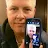Jim Whittier

I love this calculator! It has (almost) everything I would expect for a scientific graphing calculator. The only thing it doesn't do is work with hexadecimal or binary numbers but it's not very often that I need this feature.

2.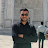Sushant Pandey

It's amazing if you are looking for an app to quickly check waveforms. And since it is from MATLAB, it's accurate and reliable.

3.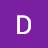Divyanshu Singh

Using experience is so simple to this aap this aap gives you graph of trigonometric ratios in 2d-3d .it has many other things for you.

4.Cabdiwali Maxamuud shire

Thank you (gc) like this app it important for student to learn some thing. it goood( 100%)look good revews only.

5.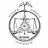Sabyasachi Pramanik

I'm a fan of this calculator. I thank the developers for adding 1day pro for each vidio

6.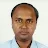Saurabh Sarkar

Great app.Has helped me in everything I did with it.

7.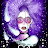Paolo Abueg

The best calculator i have ever used.

8.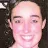maria baptista

Absolutely perfect, I can not put any fault. Thanks

9.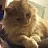Estefan Davalos

This app is a real g . Carried me through high school and is now helping me through college. I will also recommend wabbitemu calculator for a scientific calculator but this calculator can do stuff others can't. I use this for how easy it is and useful. You'll get use to it and know what I'm talking about after a while of using it.

10.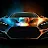reza pasban

This app very good for calculation